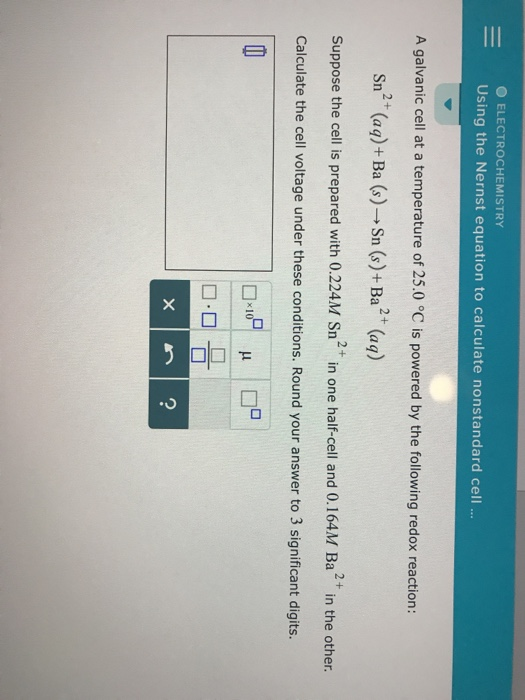# Question & Answer: A galvanic cell at a temperature of 25.0 degree C is powered by the following redox reaction:…..A galvanic cell at a temperature of 25.0 degree C is powered by the following redox reaction: Sn^2+ (aq) + Ba(s) rightarrow Sn(s) Ba^2+(aq) Suppose the cell is prepared with 0.224M Sn^2+ in one half-cell and 0.164M Ba^2+ in the other. Calculate the cell voltage under these conditions. Round your answer to 3 significant digits.